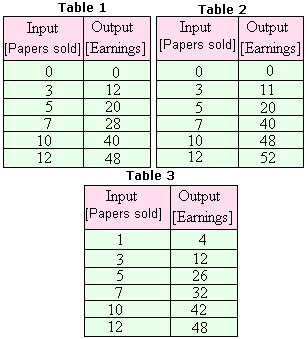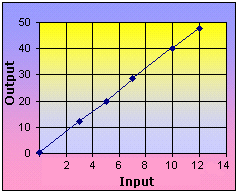# Function

## Definition Of Function

Function is a relation in which each element of the domain is paired with exactly one element of the range.

A function is a relationship between two quantities in which one quantity depends on the other.
A function is a many-to-one (or sometimes one-to-one) relation.

### Example of Function

f(x) = x + 4,, f(s) = 7s are few examples of function.

### Solved Example on Function

#### Ques: Brad sells a vacuum cleaner and earns $4 as commission for each vacuum cleaner he sells. The owner pays him depending on how many vacuum cleaners he sells. Identify the table that best suits the situation, also plot a graph for the input - output table.##### Choices: A. Table 1 B. Table 2 C. Table 3 D. None of the above Correct Answer: A ### Solution: Step 1: The commission earned by Brad on each vacuum cleaner he sells =$4.
Step 2: The amount earned by Brad (a) = 4 x number of vacuum cleaners he sells (n).
Step 3: a = 4n [Original equation.]
Step 4: a = 4(0) = 0 [Substitute 0 for n and simplify.]
Step 5: a = 4(3) = 12 [Substitute 3 for n and simplify.]
Step 6: a = 4(5) = 20 [Substitute 5 for n and simplify.]
Step 7: a = 4(7) = 28 [Substitute 7 for n and simplify.]
Step 8: a = 4(10) = 40 [Substitute 10 for n and simplify.]
Step 9: a = 4(12) = 48 [Substitute 12 for n and simplify.]
Step 10: Among the choices, Table 1 satisfies the equation.
Step 11: The graph below represents the function a = 4n.